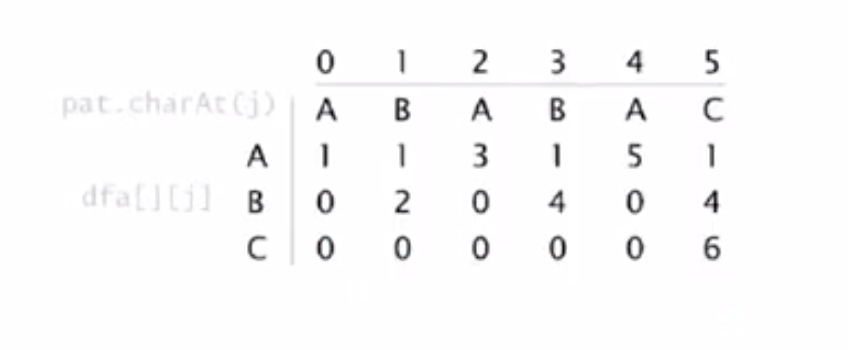# 问题描述

KMP算法，是用来解决字符串匹配问题的。字符串匹配问题，就是给定一个pattern串，一个text串，找出pattern串是否出现在text中，出现在什么位置等等。KMP算法可以在O(len(text))的时间复杂度做完。

# 思想与实现

## 有限自动机的理解方式

public KMP(char[] pattern, int R) {
this.R = R;
this.pattern = new char[pattern.length];
for (int j = 0; j < pattern.length; j++)
this.pattern[j] = pattern[j];

// build DFA from pattern
int M = pattern.length;
dfa = new int[R][M];
dfa[pattern] = 1;
for (int X = 0, j = 1; j < M; j++) {
for (int c = 0; c < R; c++)
dfa[c][j] = dfa[c][X];     // Copy mismatch cases.
dfa[pattern[j]][j] = j+1;      // Set match case.
X = dfa[pattern[j]][X];        // Update restart state.
}
}

public int search(String txt) {

// simulate operation of DFA on text
int M = pat.length();
int N = txt.length();
int i, j;
for (i = 0, j = 0; i < N && j < M; i++) {
j = dfa[txt.charAt(i)][j];
}
if (j == M) return i - M;    // found
return N;                    // not found
}构建有限自动机示例(pattern="ababac")

## 前缀后缀的理解方式

index		0	1	2	3	4
Pattern		A	B	A	B	C
next		0	0	1	2	0